### Home > PC > Chapter 1 > Lesson 1.4.2 > Problem1-154

1-154.
1. Given the graph of k(x) to the right, sketch the following functions. Homework Help ✎

1. k(x − 2) + 3

2. k(x) − 2

3.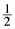k(x) + 1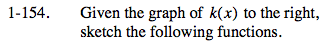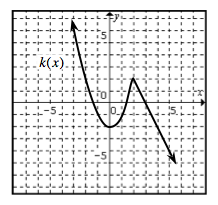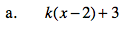Shift the curve right 2 units and up 3 units.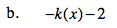Reflect the curve vertically, then shift it vertically.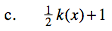Vertically "stretch" the curve by 1/2, then shift the curve vertically.